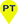€ 36.10€ 38.00
Risparmi: € 1.90 (5%)
Disponibilità immediata solo 1 pz.
Ordina entro 5 ore e 47 minuti e scegli spedizione espressa per riceverlo domani. Scopri come100% Pagamento sicuro

Ne hai uno usato?Scegli il punto di consegna
e ritira quando vuoiScopri come# Mathematical analysis tools for engineering

## di Franco Tomarelli

• Editore: Esculapio
• Data di Pubblicazione: ottobre 2019
• EAN: 9788893851619
• ISBN: 889385161X
• Pagine: 448
• Formato: brossura

### Descrizione del libro

This book is an introduction to the study of ordinary differential equations and partial differential equations, ranging from elementary techniques to advanced tools. The presentation focusses on initial value problems, boundary value problems, equations with delayed argument and analysis of periodic solutions: main goal is the analysis of diffusion equation, wave equation Laplace equation and signals. The study of relevant examples of differential models highlights the notion of well-posed problem. An expanded tutorial chapter collects the topics from basic undergraduate calculus that are used in subsequent chapters. A wide exposition concerning classical methods for solving problems related to differential equations is available: mainly separation of variables and Fourier series, with basic worked exercises. A whole chapter deals with the analytic functions of complex variable. An introduction to function spaces, distributions and basic notions of functional analysis is present. Several chapters are devoted to Fourier and Laplace transforms methods to solve boundary value problems and initial value problems for differential equations. Tools for the analysis appear gradually: first in function spaces, then in the more general framework of distributions, where a powerful arsenal of techniques allows dealing with impulsive signals and singularities in both data and solutions of differential problems.